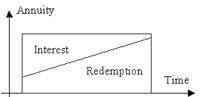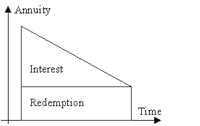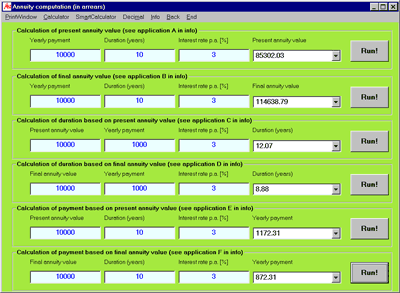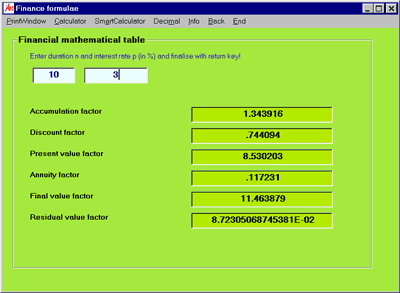# MasterAllRound: the PC calculator

## MAR FinWith MasterAllRound, interest computation, loan computation and annuity computation are readily available.

## Interest computations

You can perform the following interest computations with MasterAllRound. The input is absolutely easy and straightforward:
• Compound interest 1: calculation of final capital
Iinput is the start capital, duration and the yearly interest rate. As the result, you obtain the final capital. (example final capital computation)

• Compound interest 2: calculation of duration
Input is the start capital, the desired final capital and yearly interest rate. As the result, you obtain the necessary duration.

• Compound interest 3: calculation of interest rate
Input is the start capital, the planned final capital and the duration. As the result, you obtain the necessary yearly interest rate.

• Compound interest 4: calculation of start capital
Input is the yearly interest rate, the requested final capital and the duration. As the result, you obtain the necessary start capital.

• Daily interest: MasterAllRound computes the final capital after a specified time frame in days for a constant yearly intereste rate for your start capital

• Monthly payment: MasterAllRound computes the final capital for monthly saving rates.

• Yearly payment: MasterAllRound computes the final capital for yearly saving rates.

## Loan computations

With MasterAllRound, you can easily compare loan conditions. These calculations allows you to analyze different offers - not taking into account extra charges or special contract conditions.• Amortisation by annuities: Amortisation by annuities means that the repayment amount, that is the amount of interest and redemption, stays constant over the repayment period. Therefore, the redemption rate increases and the interest rate decreases over the years. (example loan computation)• Amortisation by instalments: Amortisation by instalments means that the redemption rate is constant over the years. The interest amount decreases over the years and therefore the repayment rate decreases accordingly.

## Annuity computationsIn finance, an annuity is a constant sum of money payable at regular intervals. All annuity computations in MasterAllRound are in arrears, that is for single payments at the end of each period. With MasterAllRound, you can compute the following calculations:
• Calculation of present annuity value
• Calculation of final annuity value
• Calculation of duration, based on present annuity value
• Calculation of duration, based on final annuity value
• Calculation of payment, based on present annuity value
• Calculation of payment, based on final annuity value

## Finance formulaeThanks to MasterAllRound, printed tables are out-of-date! MasterAllRound gives you, dependent on the number of periodic payments "n" and the compound interest rate in percent "i", the following factors:
• Accumulation factor
• Discount factor
• Present value factor
• Annuity factor
• Final value factor
• Residual value factor

MAR Cal, MAR Fin and MAR Plus are free for individuals, schools and other educational institutions. The usage of any MAR-program for commercial purpose requires the preliminarily written consent of the MAR Team.